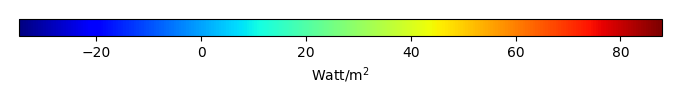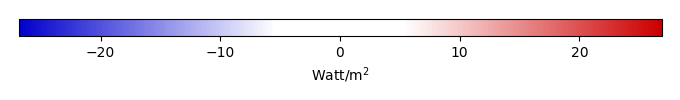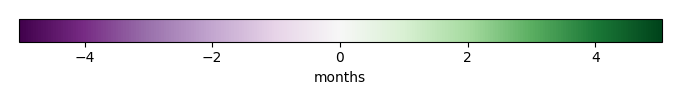# Mean State

Period Mean (original grids) [Watt/m2]
Model Period Mean (intersection) [Watt/m2]
Model Period Mean (complement) [Watt/m2]
Benchmark Period Mean (intersection) [Watt/m2]
Benchmark Period Mean (complement) [Watt/m2]
Bias [Watt/m2]
RMSE [Watt/m2]
Phase Shift [months]
Bias Score 
RMSE Score 
Seasonal Cycle Score 
Spatial Distribution Score 
Overall Score 
Benchmark [-] 44.1
CLM5PHSOFF [-] 44.7 43.8 53.4 44.2 12.4 -0.488 15.8 1.57 0.446 0.229 0.746 0.935 0.517
CLM5PHSON [-] 45.4 45.0 52.0 44.2 12.4 1.09 14.3 1.87 0.481 0.266 0.698 0.950 0.532
Period Mean (original grids) [Watt/m2]
Model Period Mean (intersection) [Watt/m2]
Model Period Mean (complement) [Watt/m2]
Benchmark Period Mean (intersection) [Watt/m2]
Benchmark Period Mean (complement) [Watt/m2]
Bias [Watt/m2]
RMSE [Watt/m2]
Phase Shift [months]
Bias Score 
RMSE Score 
Seasonal Cycle Score 
Spatial Distribution Score 
Overall Score 
Benchmark [-] 57.5
CLM5PHSOFF [-] 49.8 49.7 76.1 57.5 20.8 -8.01 19.9 0.569 0.430 0.435 0.958 0.861 0.624
CLM5PHSON [-] 50.8 50.9 67.0 57.5 20.8 -6.70 20.0 0.772 0.447 0.430 0.936 0.843 0.617
Period Mean (original grids) [Watt/m2]
Model Period Mean (intersection) [Watt/m2]
Model Period Mean (complement) [Watt/m2]
Benchmark Period Mean (intersection) [Watt/m2]
Benchmark Period Mean (complement) [Watt/m2]
Bias [Watt/m2]
RMSE [Watt/m2]
Phase Shift [months]
Bias Score 
RMSE Score 
Seasonal Cycle Score 
Spatial Distribution Score 
Overall Score 
Benchmark [-] 52.8
CLM5PHSOFF [-] 56.3 55.2 66.4 53.0 19.6 2.15 18.1 0.474 0.580 0.457 0.955 0.975 0.685
CLM5PHSON [-] 56.3 56.1 61.4 53.0 19.6 3.43 17.7 0.747 0.576 0.490 0.915 0.971 0.688
Period Mean (original grids) [Watt/m2]
Model Period Mean (intersection) [Watt/m2]
Model Period Mean (complement) [Watt/m2]
Benchmark Period Mean (intersection) [Watt/m2]
Benchmark Period Mean (complement) [Watt/m2]
Bias [Watt/m2]
RMSE [Watt/m2]
Phase Shift [months]
Bias Score 
RMSE Score 
Seasonal Cycle Score 
Spatial Distribution Score 
Overall Score 
Benchmark [-] 18.0
CLM5PHSOFF [-] 14.0 15.2 4.43 18.1 5.64 -2.93 21.7 0.726 0.772 0.455 0.940 0.920 0.709
CLM5PHSON [-] 15.6 16.0 7.19 18.1 5.64 -2.63 19.1 0.548 0.786 0.515 0.959 0.930 0.741
Period Mean (original grids) [Watt/m2]
Model Period Mean (intersection) [Watt/m2]
Model Period Mean (complement) [Watt/m2]
Benchmark Period Mean (intersection) [Watt/m2]
Benchmark Period Mean (complement) [Watt/m2]
Bias [Watt/m2]
RMSE [Watt/m2]
Phase Shift [months]
Bias Score 
RMSE Score 
Seasonal Cycle Score 
Spatial Distribution Score 
Overall Score 
Benchmark [-] 22.1
CLM5PHSOFF [-] 28.4 25.9 33.2 22.8 10.8 3.21 15.4 1.47 0.261 0.193 0.778 0.790 0.443
CLM5PHSON [-] 32.3 31.3 36.9 22.8 10.8 10.5 16.4 1.34 0.305 0.199 0.799 0.866 0.474
Period Mean (original grids) [Watt/m2]
Model Period Mean (intersection) [Watt/m2]
Model Period Mean (complement) [Watt/m2]
Benchmark Period Mean (intersection) [Watt/m2]
Benchmark Period Mean (complement) [Watt/m2]
Bias [Watt/m2]
RMSE [Watt/m2]
Phase Shift [months]
Bias Score 
RMSE Score 
Seasonal Cycle Score 
Spatial Distribution Score 
Overall Score 
Benchmark [-] 27.0
CLM5PHSOFF [-] 19.9 19.7 29.7 27.1 8.81 -7.36 18.0 0.673 0.697 0.558 0.946 0.970 0.746
CLM5PHSON [-] 20.6 20.5 27.0 27.1 8.81 -6.35 15.9 0.553 0.733 0.601 0.949 0.935 0.764
Period Mean (original grids) [Watt/m2]
Model Period Mean (intersection) [Watt/m2]
Model Period Mean (complement) [Watt/m2]
Benchmark Period Mean (intersection) [Watt/m2]
Benchmark Period Mean (complement) [Watt/m2]
Bias [Watt/m2]
RMSE [Watt/m2]
Phase Shift [months]
Bias Score 
RMSE Score 
Seasonal Cycle Score 
Spatial Distribution Score 
Overall Score 
Benchmark [-] 28.4
CLM5PHSOFF [-] 36.9 36.6 39.6 28.4 24.4 8.11 18.8 0.716 0.674 0.527 0.931 0.942 0.720
CLM5PHSON [-] 35.5 35.2 39.6 28.4 24.4 6.80 19.3 0.795 0.664 0.515 0.920 0.937 0.710
Period Mean (original grids) [Watt/m2]
Model Period Mean (intersection) [Watt/m2]
Model Period Mean (complement) [Watt/m2]
Benchmark Period Mean (intersection) [Watt/m2]
Benchmark Period Mean (complement) [Watt/m2]
Bias [Watt/m2]
RMSE [Watt/m2]
Phase Shift [months]
Bias Score 
RMSE Score 
Seasonal Cycle Score 
Spatial Distribution Score 
Overall Score 
Benchmark [-] 40.3
CLM5PHSOFF [-] 39.6 39.1 43.2 40.6 11.0 -1.62 18.8 0.606 0.542 0.493 0.946 0.856 0.666
CLM5PHSON [-] 37.6 37.1 42.1 40.6 11.0 -3.33 20.5 0.882 0.499 0.461 0.910 0.879 0.642
Period Mean (original grids) [Watt/m2]
Model Period Mean (intersection) [Watt/m2]
Model Period Mean (complement) [Watt/m2]
Benchmark Period Mean (intersection) [Watt/m2]
Benchmark Period Mean (complement) [Watt/m2]
Bias [Watt/m2]
RMSE [Watt/m2]
Phase Shift [months]
Bias Score 
RMSE Score 
Seasonal Cycle Score 
Spatial Distribution Score 
Overall Score 
Benchmark [-] 15.2
CLM5PHSOFF [-] 5.54 6.38 -2.54 15.2 3.78 -8.87 21.5 0.928 0.685 0.481 0.927 0.899 0.695
CLM5PHSON [-] 4.37 4.77 -2.26 15.2 3.78 -10.7 22.1 0.816 0.642 0.486 0.933 0.847 0.679
Period Mean (original grids) [Watt/m2]
Model Period Mean (intersection) [Watt/m2]
Model Period Mean (complement) [Watt/m2]
Benchmark Period Mean (intersection) [Watt/m2]
Benchmark Period Mean (complement) [Watt/m2]
Bias [Watt/m2]
RMSE [Watt/m2]
Phase Shift [months]
Bias Score 
RMSE Score 
Seasonal Cycle Score 
Spatial Distribution Score 
Overall Score 
Benchmark [-] 56.5
CLM5PHSOFF [-] 59.2 59.8 54.4 56.9 15.0 2.78 17.4 0.642 0.704 0.568 0.953 0.805 0.720
CLM5PHSON [-] 59.7 60.1 51.0 56.9 15.0 2.71 15.4 0.540 0.712 0.620 0.962 0.893 0.761
Period Mean (original grids) [Watt/m2]
Model Period Mean (intersection) [Watt/m2]
Model Period Mean (complement) [Watt/m2]
Benchmark Period Mean (intersection) [Watt/m2]
Benchmark Period Mean (complement) [Watt/m2]
Bias [Watt/m2]
RMSE [Watt/m2]
Phase Shift [months]
Bias Score 
RMSE Score 
Seasonal Cycle Score 
Spatial Distribution Score 
Overall Score 
Benchmark [-] 20.2
CLM5PHSOFF [-] 26.1 24.5 36.6 20.4 7.08 4.04 16.7 0.654 0.721 0.605 0.942 0.801 0.734
CLM5PHSON [-] 19.6 19.2 29.0 20.4 7.08 -0.160 16.0 0.832 0.722 0.598 0.916 0.822 0.731
Period Mean (original grids) [Watt/m2]
Model Period Mean (intersection) [Watt/m2]
Model Period Mean (complement) [Watt/m2]
Benchmark Period Mean (intersection) [Watt/m2]
Benchmark Period Mean (complement) [Watt/m2]
Bias [Watt/m2]
RMSE [Watt/m2]
Phase Shift [months]
Bias Score 
RMSE Score 
Seasonal Cycle Score 
Spatial Distribution Score 
Overall Score 
Benchmark [-] 34.3
CLM5PHSOFF [-] 31.4 36.9 13.9 34.6 11.5 2.22 19.7 0.823 0.594 0.475 0.923 0.928 0.679
CLM5PHSON [-] 31.0 36.8 6.87 34.6 11.5 2.16 19.1 0.881 0.599 0.490 0.911 0.925 0.683
Period Mean (original grids) [Watt/m2]
Model Period Mean (intersection) [Watt/m2]
Model Period Mean (complement) [Watt/m2]
Benchmark Period Mean (intersection) [Watt/m2]
Benchmark Period Mean (complement) [Watt/m2]
Bias [Watt/m2]
RMSE [Watt/m2]
Phase Shift [months]
Bias Score 
RMSE Score 
Seasonal Cycle Score 
Spatial Distribution Score 
Overall Score 
Benchmark [-] 22.7
CLM5PHSOFF [-] 47.7 49.9 45.4 22.8 18.3 26.9 36.3 1.27 0.516 0.506 0.928 0.778 0.647
CLM5PHSON [-] 45.5 48.2 43.0 22.8 18.3 26.2 36.6 1.26 0.509 0.485 0.937 0.776 0.639
Period Mean (original grids) [Watt/m2]
Model Period Mean (intersection) [Watt/m2]
Model Period Mean (complement) [Watt/m2]
Benchmark Period Mean (intersection) [Watt/m2]
Benchmark Period Mean (complement) [Watt/m2]
Bias [Watt/m2]
RMSE [Watt/m2]
Phase Shift [months]
Bias Score 
RMSE Score 
Seasonal Cycle Score 
Spatial Distribution Score 
Overall Score 
Benchmark [-] 17.0
CLM5PHSOFF [-] 8.63 9.84 0.308 17.0 4.51 -7.27 21.7 1.03 0.719 0.492 0.905 0.900 0.702
CLM5PHSON [-] 9.34 9.87 0.733 17.0 4.51 -7.63 21.0 0.966 0.705 0.511 0.907 0.884 0.704
Period Mean (original grids) [Watt/m2]
Model Period Mean (intersection) [Watt/m2]
Model Period Mean (complement) [Watt/m2]
Benchmark Period Mean (intersection) [Watt/m2]
Benchmark Period Mean (complement) [Watt/m2]
Bias [Watt/m2]
RMSE [Watt/m2]
Phase Shift [months]
Bias Score 
RMSE Score 
Seasonal Cycle Score 
Spatial Distribution Score 
Overall Score 
Benchmark [-] 47.7
CLM5PHSOFF [-] 50.0 49.2 54.6 48.2 15.9 0.827 22.1 0.647 0.500 0.524 0.937 0.908 0.679
CLM5PHSON [-] 50.2 49.8 55.9 48.2 15.9 1.46 21.3 0.676 0.515 0.534 0.932 0.885 0.680
Period Mean (original grids) [Watt/m2]
Model Period Mean (intersection) [Watt/m2]
Model Period Mean (complement) [Watt/m2]
Benchmark Period Mean (intersection) [Watt/m2]
Benchmark Period Mean (complement) [Watt/m2]
Bias [Watt/m2]
RMSE [Watt/m2]
Phase Shift [months]
Bias Score 
RMSE Score 
Seasonal Cycle Score 
Spatial Distribution Score 
Overall Score 
Benchmark [-] 28.6
CLM5PHSOFF [-] 34.0 33.6 39.2 28.7 12.1 4.78 14.8 0.837 0.700 0.474 0.898 0.971 0.704
CLM5PHSON [-] 30.5 30.4 32.8 28.7 12.1 1.84 14.4 0.637 0.727 0.471 0.933 0.981 0.717
Period Mean (original grids) [Watt/m2]
Model Period Mean (intersection) [Watt/m2]
Model Period Mean (complement) [Watt/m2]
Benchmark Period Mean (intersection) [Watt/m2]
Benchmark Period Mean (complement) [Watt/m2]
Bias [Watt/m2]
RMSE [Watt/m2]
Phase Shift [months]
Bias Score 
RMSE Score 
Seasonal Cycle Score 
Spatial Distribution Score 
Overall Score 
Benchmark [-] 26.7
CLM5PHSOFF [-] 25.3 25.2 30.4 26.9 8.98 -1.75 17.3 0.672 0.776 0.573 0.947 0.965 0.767
CLM5PHSON [-] 24.9 24.9 24.5 26.9 8.98 -1.95 16.2 0.693 0.785 0.593 0.937 0.969 0.775
Period Mean (original grids) [Watt/m2]
Model Period Mean (intersection) [Watt/m2]
Model Period Mean (complement) [Watt/m2]
Benchmark Period Mean (intersection) [Watt/m2]
Benchmark Period Mean (complement) [Watt/m2]
Bias [Watt/m2]
RMSE [Watt/m2]
Phase Shift [months]
Bias Score 
RMSE Score 
Seasonal Cycle Score 
Spatial Distribution Score 
Overall Score 
Benchmark [-] 49.7
CLM5PHSOFF [-] 45.2 44.7 64.5 49.8 11.0 -5.25 17.3 0.836 0.725 0.642 0.921 0.851 0.756
CLM5PHSON [-] 44.9 44.6 66.6 49.8 11.0 -5.13 17.0 0.824 0.726 0.646 0.923 0.891 0.766
Period Mean (original grids) [Watt/m2]
Model Period Mean (intersection) [Watt/m2]
Model Period Mean (complement) [Watt/m2]
Benchmark Period Mean (intersection) [Watt/m2]
Benchmark Period Mean (complement) [Watt/m2]
Bias [Watt/m2]
RMSE [Watt/m2]
Phase Shift [months]
Bias Score 
RMSE Score 
Seasonal Cycle Score 
Spatial Distribution Score 
Overall Score 
Benchmark [-] 30.2
CLM5PHSOFF [-] 28.6 28.2 43.7 30.3 13.0 -2.05 14.4 1.30 0.412 0.225 0.833 0.619 0.463
CLM5PHSON [-] 30.7 30.7 45.7 30.3 13.0 0.943 12.6 1.42 0.427 0.277 0.810 0.701 0.498
Period Mean (original grids) [Watt/m2]
Model Period Mean (intersection) [Watt/m2]
Model Period Mean (complement) [Watt/m2]
Benchmark Period Mean (intersection) [Watt/m2]
Benchmark Period Mean (complement) [Watt/m2]
Bias [Watt/m2]
RMSE [Watt/m2]
Phase Shift [months]
Bias Score 
RMSE Score 
Seasonal Cycle Score 
Spatial Distribution Score 
Overall Score 
Benchmark [-] 40.3
CLM5PHSOFF [-] 43.4 43.5 44.6 40.4 9.36 3.06 15.9 0.765 0.596 0.392 0.924 0.891 0.639
CLM5PHSON [-] 42.8 43.1 40.0 40.4 9.36 2.70 14.4 0.875 0.607 0.430 0.911 0.875 0.651
Period Mean (original grids) [Watt/m2]
Model Period Mean (intersection) [Watt/m2]
Model Period Mean (complement) [Watt/m2]
Benchmark Period Mean (intersection) [Watt/m2]
Benchmark Period Mean (complement) [Watt/m2]
Bias [Watt/m2]
RMSE [Watt/m2]
Phase Shift [months]
Bias Score 
RMSE Score 
Seasonal Cycle Score 
Spatial Distribution Score 
Overall Score 
Benchmark [-] 41.5
CLM5PHSOFF [-] 64.8 62.8 73.4 42.0 6.56 20.3 33.8 0.554 0.491 0.535 0.954 0.851 0.673
CLM5PHSON [-] 62.7 62.0 68.8 42.0 6.56 18.5 33.8 0.746 0.500 0.512 0.939 0.830 0.659

# Temporally integrated period mean

BENCHMARK MEANMODEL MEANBIASBIAS SCORERMSERMSE SCOREBENCHMARK MAX MONTHMODEL MAX MONTHDIFFERENCE IN MAX MONTHSEASONAL CYCLE SCORESPATIAL TAYLOR DIAGRAMMODEL COLORS# Spatially integrated regional mean

MODEL COLORSREGIONAL MEANANNUAL CYCLEMONTHLY ANOMALYANNUAL CYCLE# All Models

BenchmarkCLM5PHSOFFCLM5PHSON# Data Information

creation_date: Tue Jul 28 15:54:11 PDT 2015

source_file: This product is generated from monthly Global Biosphere Amtospher Flux (GBAF) observations

title: derived GBAF in 0.5x0.5

Approach: I read this variable from data file, and then save the data in NetCDF format by each month and each year. Meanwhile, I also converted the unit from MJoule*m-2*d-1 to Watt/m2.

Temporal resolution: monthly

General information: This product was derived from Monthly estimates of global biosphere-atmosphere fluxes from Biogeochemistry group at Max Planck Institute in Jena, Germany.

Spatial resolution: 0.5x0.5 degree